Author: Oscar Cronquist Article last updated on May 06, 2022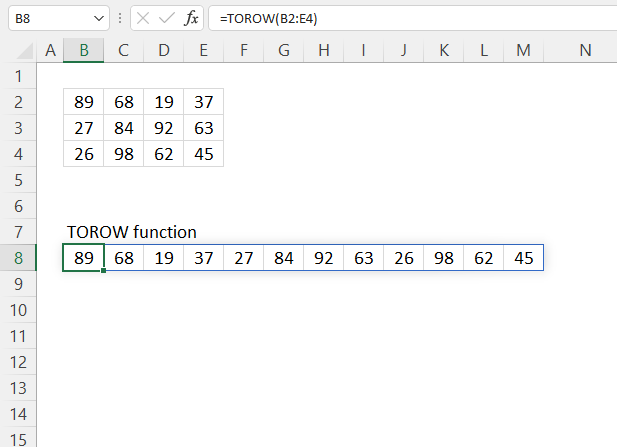The TOROW function rearranges values from a 2D cell range to a single row.

Dynamic array formula in cell B8:

=TOROW(B2:E4)

The TOROW function is available to Excel 365 users and is in the "Array manipulation" category. A dynamic array formula is entered as a regular formula, however, it spills array values automatically to adjacent cells below or to the right or both.

## 1. TOROW Function Syntax

TOROW(array, [ignore], [scan_by_col])

## 2. TOROW Function Arguments

 array Required. The source cell range or array. [ignore] Optional. Ignore specified values. 0 - keep all values (default) 1 - ignore blanks 2 - ignore errors 3- ignore blanks and errors [scan_by_col] Optional. How the function fetches the values from the source. FALSE - by row (default). TRUE - by column.

## 3. TOROW Function example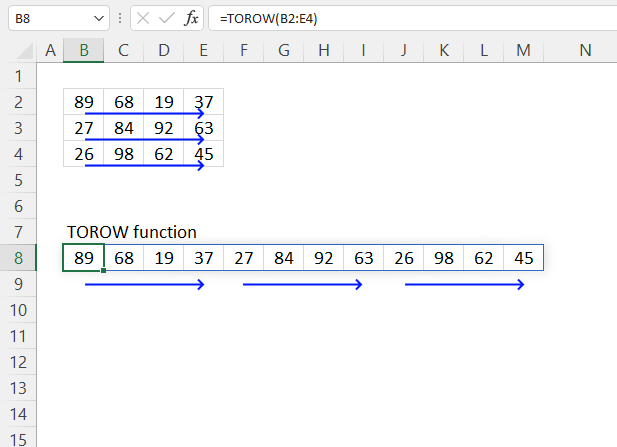The graphic above shows how the TOROW function rearranges values to fit a single row; in its default form, it obtains values by row.

Dynamic array formula in cell B8:

=TOROW(B2:E4)
It's worth noting that if any of the values in the source range change, the values in the TOROW function output will change as well.

### 3.1 Explaining formula

#### Step 1 - TOROW function

TOROW(array, [ignore], [scan_by_col])

array - B2:E4

#### Step 3 - Evaluate function

TOROW(B2:E4)

becomes

TOROW({89, 68, 19, 37;
27, 84, 92, 63;
26, 98, 62, 45})

and returns

{89; 68; 19; 37; 27; 84; 92; 63; 26; 98; 62; 45}

## 4. TOROW Function example - by column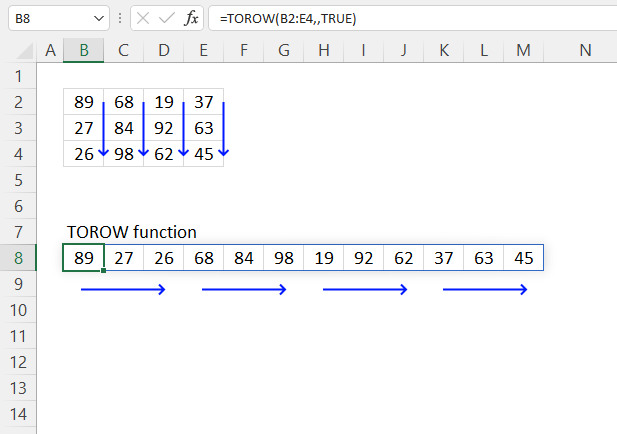The picture above demonstrates how the TOROW function rearranges the values to fit a  single row, this example shows it fetching values column by column. Default value is FALSE - by row.

Dynamic array formula in cell B8:

=TOROW(B2:E4, , TRUE)

### 4.1 Explaining formula

#### Step 1 - TOROW function

TOROW(array, [ignore], [scan_by_col])

#### Step 2 - Populate arguments

array - B2:E4
[scan_by_col]) - TRUE

#### Step 3 - Evaluate function

TOROW(B2:E4, , TRUE)

becomes

TOROW({89, 68, 19, 37;
27, 84, 92, 63;
26, 98, 62, 100})

and returns

{89, 27, 26, 68, 84, 98, 19, 92, 62, 37, 63, 45}

The comma is a column delimiting character, the semicolon is a row delimiting character. You may have other delimiting characters, it depends on your regional settings.

Which delimiting values do I use?

• Double press with left mouse button on an empty cell.
• Type = (equal sign).
• Select a cell range containing two rows and two columns. They can be empty, it doesn't matter.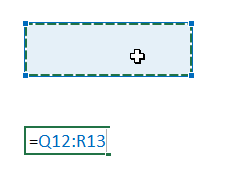• Press F9 to convert the cell reference to constants.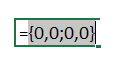• The array that appears shows your delimiting characters.

## 5. TOROW Function - blanks and errors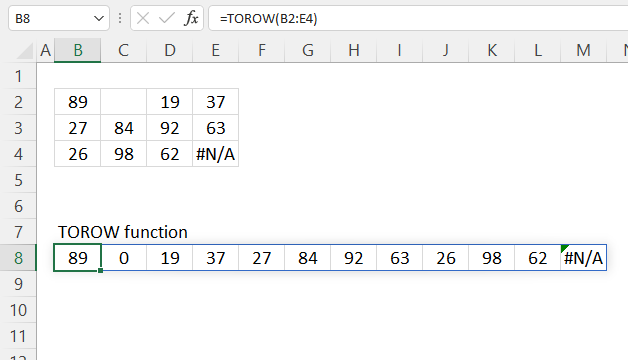The image above demonstrates what happens when your source data has empty values and errors.

The result is a horizontal array containing a 0 (zero) located at the empty values and the error values are kept.

Dynamic array formula in cell B8:

=TOROW(B2:E4)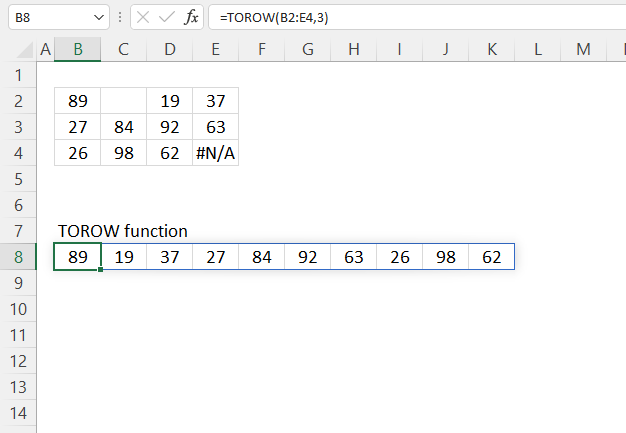The picture above shows how to handle blanks and error values. The second argument lets you determine the outcome for blank and error values.

Here are all valid numbers for the second argument:
0 - keep all values (default)
1 - ignore blanks
2 - ignore errors
3- ignore blanks and errors

Dynamic array formula in cell B8:

=TOROW(B2:E4, 3)

### 5.1 Explaining formula

TOROW(array, [ignore], [scan_by_col])

TOROW(B2:E4, 3)

becomes

TOROW({89, "", 19, 37;
27, 0, 92, 63;
26, 98, 62, #N/A}
)

and returns

{89, 19, 37, 27, 84, 92, 63, 26, 98, 62}

## 6. TOROW function alternative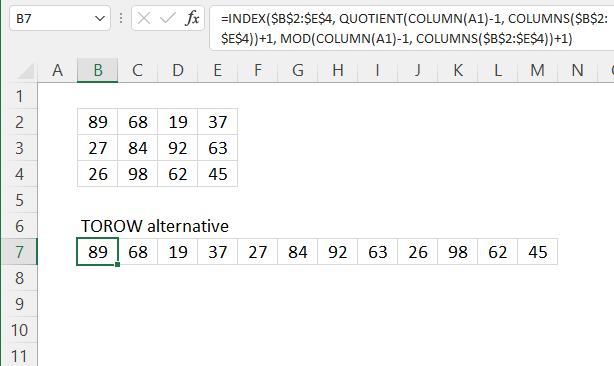The following formula rearranges values so they fit a single column, however, the formula doesn't return an array of values. It extracts each value one by one using relative cell references.

You need to copy the cell and paste as far as needed to the right. This formula works in most Excel versions.

Formula in cell B7:

=INDEX(\$B\$2:\$E\$4, QUOTIENT(COLUMN(A1)-1, COLUMNS(\$B\$2:\$E\$4))+1, MOD(COLUMN(A1)-1, COLUMNS(\$B\$2:\$E\$4))+1)

### 6.1 Explaining formula

#### Step 1 - Calculate the number of columns in a given cell range

The COLUMNS function returns the number of columns in a given cell range.

COLUMNS(array)

COLUMNS(\$B\$2:\$E\$4)

returns 4.

#### Step 2 - Calculate column number of relative cell reference

The COLUMN function returns the column number of the top-left cell of a cell reference.

COLUMN(reference)

COLUMN(A1)-1

becomes

1-1 equals 0 (zero)

#### Step 3 - Calculate row number

The QUOTIENT function returns the integer portion of a division.

QUOTIENT(numeratordenominator)

QUOTIENT(COLUMN(A1)-1, COLUMNS(\$B\$2:\$E\$4))+1

becomes

QUOTIENT(0, 4)+1

becomes

0+1 equals 1.

#### Step 4 - Calculate column number

The MOD function returns the remainder after a number is divided by a divisor.

MOD(numberdivisor)

MOD(COLUMN(A1)-1, COLUMNS(\$B\$2:\$E\$4))+1

becomes

MOD(0, 4)+1

becomes

0 + 1 equals 1.

#### Step 5 - Get value

The INDEX function returns a value from a cell range, you specify which value based on a row and column number.

INDEX(array[row_num][column_num], [area_num])

INDEX(\$B\$2:\$E\$4, QUOTIENT(COLUMN(A1)-1, COLUMNS(\$B\$2:\$E\$4))+1, MOD(COLUMN(A1)-1, COLUMNS(\$B\$2:\$E\$4))+1)

becomes

INDEX(\$B\$2:\$E\$4, 1, 1)

and returns 89 in cell B7.

## 7. TOROW function - values in random order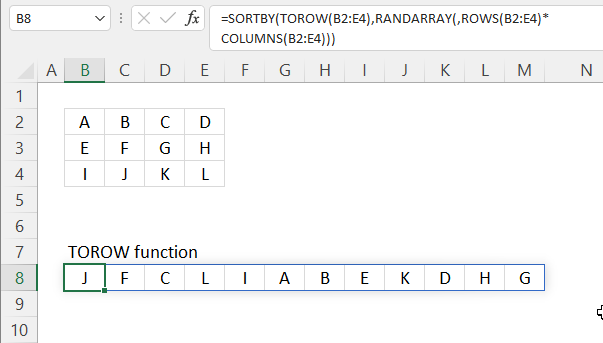The image above shows a formula that returns values to a single row in random order.

Dynamic array formula in cell B8:

=SORTBY(TOROW(B2:E4), RANDARRAY(, ROWS(B2:E4)*COLUMNS(B2:E4)))

### Explaining formula in cell B8

#### Step 1 - Calculate the number of rows in cell ref

The ROWS function returns the number of rows in a given cell range.

ROWS(array)

ROWS(B2:E4)

returns 3.

#### Step 2 - Calculate the number of columns in cell ref

The COLUMNS function returns the number of columns in a given cell range.

COLUMNS(array)

COLUMNS(B2:E4)

returns 4.

#### Step 3 - Calculate the number of cells in cell ref

The asterisk lets you multiply numbers in an Excel formula.

ROWS(B2:E4)*COLUMNS(B2:E4)

becomes

3*4 equals 12.

#### Step 4 - Create random values

The RANDARRAY function returns a table of random numbers across rows and columns.

RANDARRAY([rows], [columns], [min], [max], [whole_number])

RANDARRAY(, ROWS(B2:E4)*COLUMNS(B2:E4))

becomes

RANDARRAY(, 12)

and returns 12 random decimal values:

{0.464680665246457, 0.768212043461448, ... ,0.281771048521311}

#### Step 5 - Rearrange values

TOROW(B2:E4)

returns

{"A", "B", "C", "D", "E", "F", "G", "H", "I", "J", "K", "L"}.

#### Step 6 - Sort values in random order

The SORTBY function allows you to sort values from a cell range or array based on a corresponding cell range or array.

SORTBY(array, by_array1, [sort_order1], [by_array2, sort_order2],…)

SORTBY(TOROW(B2:E4), RANDARRAY(, ROWS(B2:E4)*COLUMNS(B2:E4)))

becomes

SORTBY({"A", "B", "C", "D", "E", "F", "G", "H", "I", "J", "K", "L"}, {0.464680665246457, 0.768212043461448, ... ,0.281771048521311})

and returns

{"H", "E", "I", "F", "L", "B", "D", "A", "J", "K", "G", "C"}. Press F9 to recalcualte values, this creates a new random order.